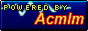Views: 11,197,562 Home | Forums | Uploader | Wiki | Object databases | IRC Rules/FAQ | Memberlist | Calendar | Stats | Online users | Last posts | Search 09-19-20 06:46 PM Guest:

 0 users reading Need help converting triangle formats! | 1 bot

 Main - Misc. ROM hacking - Need help converting triangle formats! New reply

Yoshimaster96
 Posted on 01-23-15 09:28 AM Link | #55043
GMA files store the vertices of a triangle as 3 XYZ coordinates. But for collision, LZ files store the vertices as follows:

Posted by LZ.TXT
(ROTATION IS Z THEN X THEN Y)

04 X1
04 Y1
04 Z1
04 Normal X
04 Normal Y
04 Normal Z
02 X Angle
02 Y Angle
02 Z Angle
02 Zero
04 DX2X1
04 DY2Y1
04 DX3X1
04 DY3Y1
04 ?
04 ?
04 ?
04 ?

For clarification, first coordinate is (x1,y1,z1), second coordinate is calculated as (x1+dx2x1,y1+dy2y1,z1), rotated about first point by x angle, y angle, and z angle, in the order specified above. Third coordinate is calculated similarly, from (x1+dx3x1,y1+dy3y1,z1), rotated by the same angles. The ?s are unknown, but I think they're tangent and bitangent/binormal. So how would I convert from GMA triangle to LZ triangle?

Yoshimaster96smwc
Some layout tips/code!

-Yoshimaster96

blank
 Posted on 01-24-15 04:21 AM Link | #55104
This is python code that converts a triangle given by the vertices a, b and c:

def normalize(v):
return v/numpy.linalg.norm(v)

def hat(v):
return numpy.array([-v,v,0],numpy.float32)

def reverse_angle(c,s):
if abs(c) < abs(s):
a = degrees(acos(c))
if s < 0: a = -a
else:
a = degrees(asin(s))
if c < 0: a = 180 - a
if a < 0:
if a > -0.001:
a = 0
else:
a += 360
return a

vertex = a
normal = normalize(numpy.cross(normalize(b - a),normalize(c - a)))

l = sqrt(normal*normal + normal*normal)
if abs(l) < 0.001:
if cy < 0: continue
cy = 1
sy = 0
else:
cy = normal/l
sy = normal/l

cx = l
sx = -normal

Rx = numpy.array([
[1,0,0],
[0,cx,sx],
[0,-sx,cx]],numpy.float32)

Ry = numpy.array([
[cy,0,-sy],
[0,1,0],
[sy,0,cy]],numpy.float32)

v0 = numpy.dot(Rx,numpy.dot(Ry,b - a))
l = sqrt(v0*v0 + v0*v0)
cz = v0/l
sz = v0/l

Rz = numpy.array([
[cz,sz,0],
[-sz,cz,0],
[0,0,1]],numpy.float32)

v0 = numpy.dot(Rz,v0)
v1 = numpy.dot(Rz,numpy.dot(Rx,numpy.dot(Ry,c - a)))

n0 = normalize(hat(v1 - v0))
n1 = normalize(hat(-v1))

rotation_x = reverse_angle(cx,sx)
rotation_y = reverse_angle(cy,sy)
rotation_z = reverse_angle(cz,sz)

Here v0 and v1 corresponds to your (dx2x1,dy2y1) and (dx3x1,dy3y1) and n0 and n1 corresponds to your unknowns.

Also, have you noticed that the collision is divided into a grid?

Yoshimaster96
 Posted on 01-25-15 02:02 PM Link | #55191
Posted by blank
This is python code that converts a triangle given by the vertices a, b and c:

def normalize(v):
return v/numpy.linalg.norm(v)

def hat(v):
return numpy.array([-v,v,0],numpy.float32)

def reverse_angle(c,s):
if abs(c) < abs(s):
a = degrees(acos(c))
if s < 0: a = -a
else:
a = degrees(asin(s))
if c < 0: a = 180 - a
if a < 0:
if a > -0.001:
a = 0
else:
a += 360
return a

vertex = a
normal = normalize(numpy.cross(normalize(b - a),normalize(c - a)))

l = sqrt(normal*normal + normal*normal)
if abs(l) < 0.001:
if cy < 0: continue
cy = 1
sy = 0
else:
cy = normal/l
sy = normal/l

cx = l
sx = -normal

Rx = numpy.array([
[1,0,0],
[0,cx,sx],
[0,-sx,cx]],numpy.float32)

Ry = numpy.array([
[cy,0,-sy],
[0,1,0],
[sy,0,cy]],numpy.float32)

v0 = numpy.dot(Rx,numpy.dot(Ry,b - a))
l = sqrt(v0*v0 + v0*v0)
cz = v0/l
sz = v0/l

Rz = numpy.array([
[cz,sz,0],
[-sz,cz,0],
[0,0,1]],numpy.float32)

v0 = numpy.dot(Rz,v0)
v1 = numpy.dot(Rz,numpy.dot(Rx,numpy.dot(Ry,c - a)))

n0 = normalize(hat(v1 - v0))
n1 = normalize(hat(-v1))

rotation_x = reverse_angle(cx,sx)
rotation_y = reverse_angle(cy,sy)
rotation_z = reverse_angle(cz,sz)

Here v0 and v1 corresponds to your (dx2x1,dy2y1) and (dx3x1,dy3y1) and n0 and n1 corresponds to your unknowns.

Also, have you noticed that the collision is divided into a grid?

Tried to convert this to C# (as that is the language of my program), but I got stuck. How are cx, cy, cz, sx, sy, and sz defined?

Yoshimaster96smwc
Some layout tips/code!

-Yoshimaster96

blank
 Posted on 01-26-15 12:18 AM Link | #55219
cx, sx, cy, sy, cz and sz are floats and are the cosine and sine of the rotation angles.

Yoshimaster96
 Posted on 01-26-15 05:52 AM Link | #55222
Posted by blank
cx, sx, cy, sy, cz and sz are floats and are the cosine and sine of the rotation angles.
Those aren't given however. I'm trying to find the rotation angles.

Yoshimaster96smwc
Some layout tips/code!

-Yoshimaster96

blank
 Posted on 01-26-15 07:14 AM Link | #55223
You calculate the angles using cx, sx, etc. In my code snippet this is done with the reverse_angle function.

Yoshimaster96
 Posted on 01-27-15 08:10 AM Link | #55326
You don't get it? I'm given coordinates, and am trying to find angles. I can't find sine and cosine of angles I don't know.

Yoshimaster96smwc
Some layout tips/code!

-Yoshimaster96

Kinnay
 Posted on 01-27-15 09:14 AM Link | #55328
Hmm, not sure if that's what you meant, but you can find angles in a triangle if you know the length of the sides with the cosine rule. You can easily calculate the lengths of the sides if you know the coordinates.

blank
 Posted on 01-27-15 09:39 AM Link | #55329
The code specifically shows how to calculate cx, sx, etc. without first calculating the angles.

 Main - Misc. ROM hacking - Need help converting triangle formats! New reply

 Page rendered in 0.022 seconds. (2048KB of memory used) MySQL - queries: 26, rows: 120/120, time: 0.017 seconds.Acmlmboard 2.064 (2018-07-20) © 2005-2008 Acmlm, Xkeeper, blackhole89 et al.